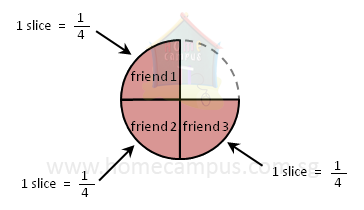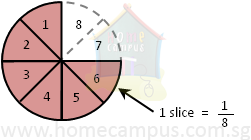## How to Divide Proper Fractions by Whole Number?

Practice Unlimited Questions

#### 2. 3 4 of a pizza is divided equally among 3 friends. What is the share of each friend?3 4
÷  3

 3 4
÷
 3 1

 31 4
×
 1 13

 1 x 1 4 x 1

 1 4

 1 4
of the pizza.
Dividing by 3 or
 3 1
is the same as multiplying by
 1 3
.

#### 3. 3 4 of a pizza is divided equally among 6 friends. What is the share of each friend?3 4
÷  6

 3 4
÷
 6 1

 31 4
×
 1 26

 1 x 1 4 x 2

 1 8